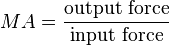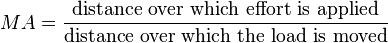# Mechanical advantage facts for kids

Kids Encyclopedia Facts

Mechanical advantage (also written as MA in formulas) is the factor by which a machine multiplies force. The mechanical advantage of a machine can be used to find out how well a machine works and whether it can perform a particular job.

The mechanical advantage of a machine is the ratio of the load (the resistance overcome by a machine) to the effort (the force applied).$MA = \frac{\text{output force}}{\text{input force}}$

For an ideal (without friction) mechanism, it is also equal to:$MA = \frac{\text{distance over which effort is applied}}{\text{distance over which the load is moved}}$

There is no unit for mechanical advantages since the unit for both input and output forces cancel out.

## Images for kidsMechanical advantage Facts for Kids. Kiddle Encyclopedia.